Examples

Chapter 11 Class 11 - Intro to Three Dimensional Geometry
Serial order wise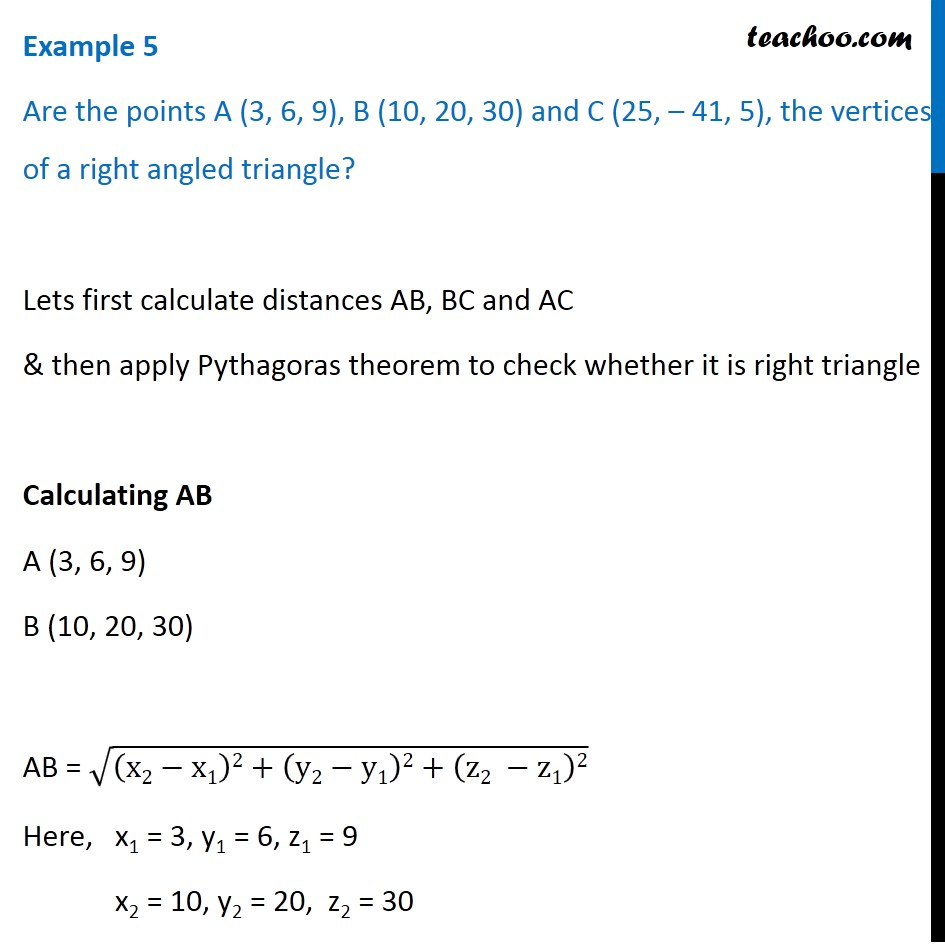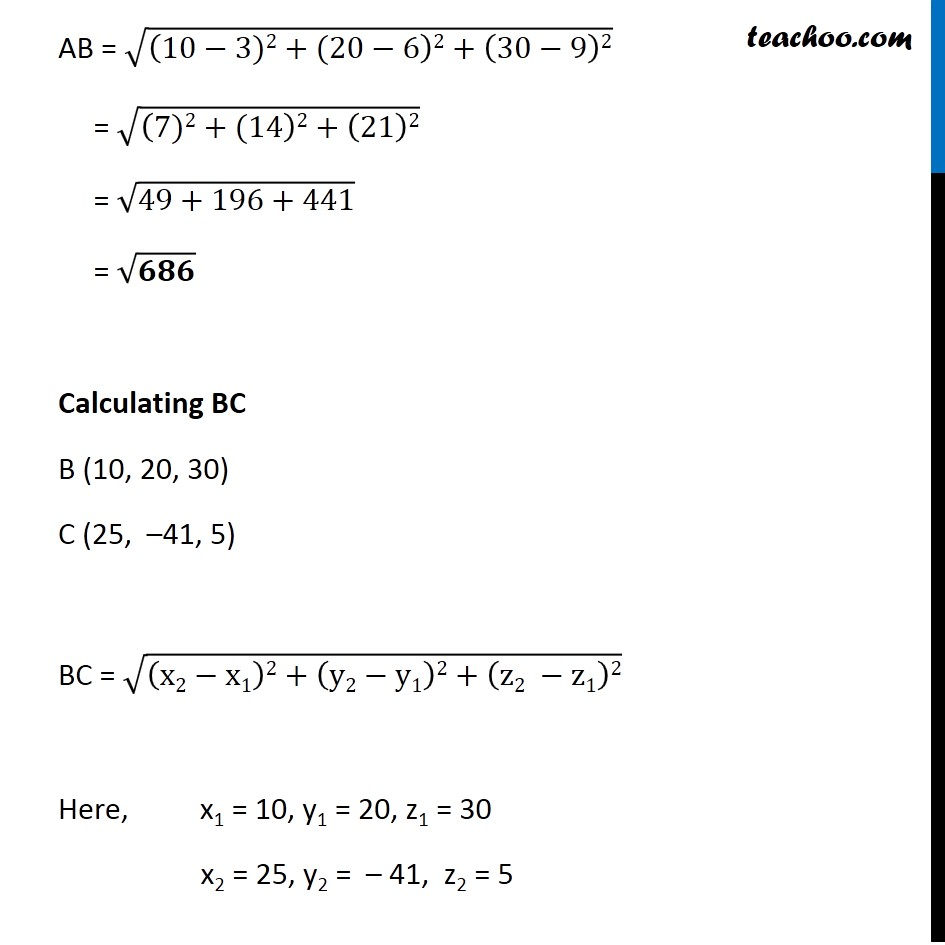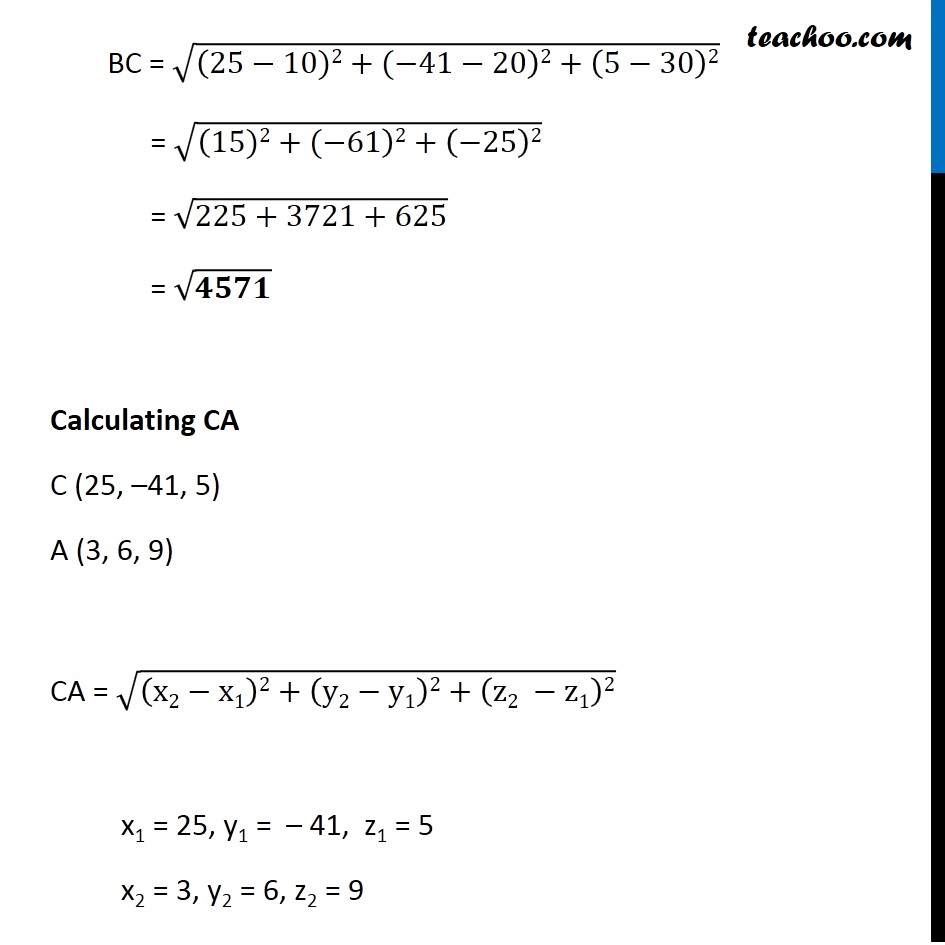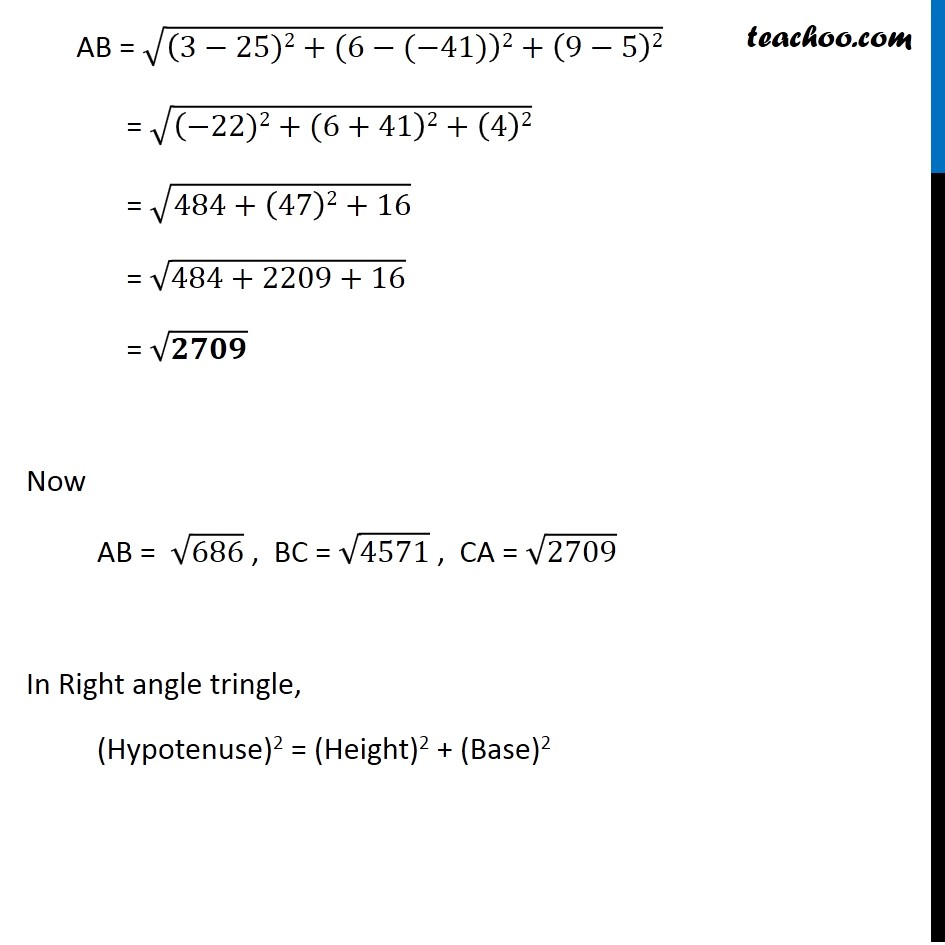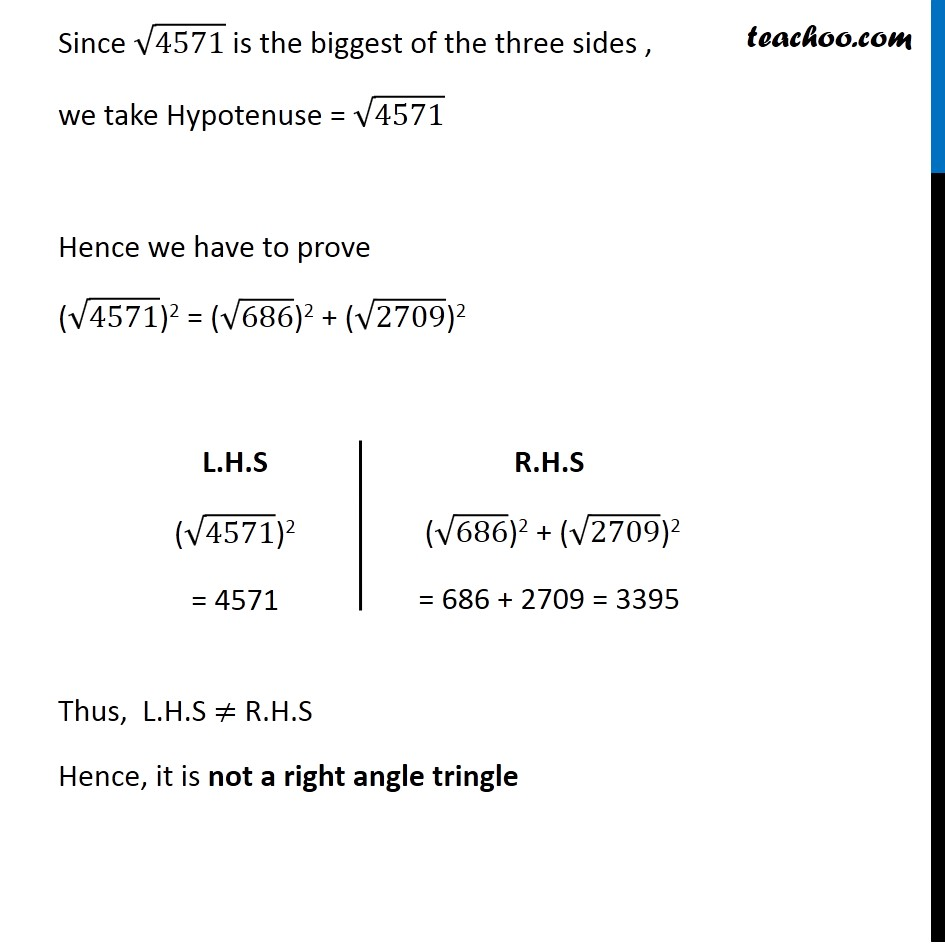Learn in your speed, with individual attention - Teachoo Maths 1-on-1 Class

### Transcript

Example 5 Are the points A (3, 6, 9), B (10, 20, 30) and C (25, – 41, 5), the vertices of a right angled triangle? Lets first calculate distances AB, BC and AC & then apply Pythagoras theorem to check whether it is right triangle Calculating AB A (3, 6, 9) B (10, 20, 30) AB = √((x2−x1)2+(y2−y1)2+(z2 −z1)2) Here, x1 = 3, y1 = 6, z1 = 9 x2 = 10, y2 = 20, z2 = 30 AB = √((10−3)2+(20−6)2+(30−9)2) = √((7)2+(14)2+(21)2) = √(49+196+441) = √𝟔𝟖𝟔 Calculating BC B (10, 20, 30) C (25, –41, 5) BC = √((x2−x1)2+(y2−y1)2+(z2 −z1)2) Here, x1 = 10, y1 = 20, z1 = 30 x2 = 25, y2 = – 41, z2 = 5 BC = √((25−10)2+(−41−20)2+(5−30)2) = √((15)2+(−61)2+(−25)2) = √(225+3721+625) = √𝟒𝟓𝟕𝟏 Calculating CA C (25, –41, 5) A (3, 6, 9) CA = √((x2−x1)2+(y2−y1)2+(z2 −z1)2) x1 = 25, y1 = – 41, z1 = 5 x2 = 3, y2 = 6, z2 = 9 AB = √((3−25)2+(6−(−41))2+(9−5)2) = √((−22)2+(6+41)2+(4)2) = √(484+(47)2+16) = √(484+2209+16) = √𝟐𝟕𝟎𝟗 Now AB = √686 , BC = √4571 , CA = √2709 In Right angle tringle, (Hypotenuse)2 = (Height)2 + (Base)2 Since √4571 is the biggest of the three sides , we take Hypotenuse = √4571 Hence we have to prove (√4571)2 = (√686)2 + (√2709)2 Thus, L.H.S ≠ R.H.S Hence, it is not a right angle tringle L.H.S (√4571)2 = 4571 R.H.S (√686)2 + (√2709)2 = 686 + 2709 = 3395